• 首先，傅里叶分析是指把一个周期或非周期函数展开成一个个三角函数的叠加，如果是对其还没有基本概念的，可以看看傅里叶分析之掐死教程，这篇文章不依赖数学公式却又十分透彻地讲述了傅里叶分析的基本概念，十分值得...
首先，傅里叶分析是指把一个周期或非周期函数展开成一个个三角函数的叠加，如果是对其还没有基本概念的，可以看看傅里叶分析之掐死教程，这篇文章不依赖数学公式却又十分透彻地讲述了傅里叶分析的基本概念，十分值得一读。但如果先深入探讨其中的数学由来，接下来会讲述详细的数学推导。
傅里叶级数
三角函数系的正交性
三角函数系：{1,sinx,cosx,sin2x,cos2x,…,sinnx,cosnx,…}，它由无数个sinnx和cosnx组成，其中n=0,1,2,…。
正交性：
$\int^\pi_{-\pi}\sin nx \cos mx dx = 0$
$\int^\pi_{-\pi}\cos nx \cos mx dx = 0，n\neq m$
$\int^\pi_{-\pi}\sin nx \sin mx dx = 0，n\neq m$
当向量点乘等于0：
$\vec a \cdot \vec b = 0$
则两个向量正交。
拓展到函数中，两个函数相乘，原本点乘的加和变成取积分，则：
$\int^{x_1}_{x_2}f(x) g(x) dx = 0$
称为两个函数正交。
证明其正交性，可以用积化和差公式：
$\int^\pi_{-\pi}\cos nx \cos mx dx = \frac{1}{2}\int^\pi_{-\pi}[cos(n-m)x+cos(n+m)x]dx =0$
其他情况同理。
周期为2π的函数展开
因此当一个函数f(x)周期为2π时，可以展开成
$f(x) = \sum^\infty_{n=0}a_ncosnx + \sum^\infty_{n=0}b_nsinnx = \frac 1 2 a_0 + \sum^\infty_{n=1}({a_ncosnx + b_nsinnx})$
对两边取积分，由于三角函数的正交性
$\int_{-\pi}^\pi f(x)dx = \frac1 2\int_{-\pi}^\pi a_0dx = \pi a_0$
$a_0 = \frac 1 {\pi}\int_{-\pi}^\pi f(x)dx$
原式乘以cos mx 再对两边取积分可得：
$\int^\pi_{-\pi}f(x) \cos mx dx = \sum^\infty_{n=1}\int^\pi_{-\pi} a_ncosnx cosmx dx = \int^\pi_{-\pi} a_ncos^2 nx dx = a_n\pi$
$a_n = \frac 1 \pi \int^\pi_{-\pi}f(x) \cos nx dx$
同理，两边同乘sinmx再取积分可得：
$b_n = \frac 1 \pi \int^\pi_{-\pi}f(x) \sin nx dx$
周期为2L的函数展开
利用换元的方法，令：$x = \frac \pi L t$ ，即$t = \frac L \pi x$，可得：
$f(t) = \frac {a_0} 2 + \sum^\infty_{n=1}({a_ncos \frac{n\pi}{L}t + b_nsin\frac{n\pi}{L}t})$
$a_0 = \frac 1 {L}\int_{-L}^L f(t)dt$
$a_n = \frac 1 L \int^L_{-L}f(x) \cos nx dx$
$b_n = \frac 1 L \int^L_{-L}f(x) \sin nx dx$
在工程中t总是从0开始，周期T=2L，ω = $\frac \pi L = \frac 2\pi T$，此时：
$f(t) = \frac {a_0} 2 + \sum^\infty_{n=1}({a_ncosωt + b_nsinωt})$
$a_0 = \frac 2 {T}\int_{0}^T f(t)dt$
$a_n = \frac 2 T \int^T_{0}f(x) \cos nx dx$
$b_n = \frac 2 T \int^T_{0}f(x) \sin nx dx$
傅里叶级数的复数表达形式
欧拉公式：$e^{iθ} = cosθ + isinθ$
可得：
$cosθ = \frac 1 2 (e^{iθ}+e^{-iθ})$
$sinθ = \frac 1 2 i(e^{iθ}-e^{-iθ})$
把这两条式子代入f(t)的式子中可得：
$f(t) = \frac {a_0} 2 + \sum_{n=1}^\infty \frac {a_n-ib_n} 2 e^{in\omega t}+ \sum_{n=1}^\infty \frac {a_n+ib_n} 2 e^{-in\omega t} = \sum_{n=0}^0 \frac {a_0} 2 e^{in\omega t} + \sum_{n=1}^\infty \frac {a_n-ib_n} 2 e^{in\omega t}+ \sum_{n=-1}^{-\infty} \frac {a_{-n}+ib_{-n}} 2 e^{in\omega t} = \sum_{-\infty}^\infty C_n e^{in\omega t}$
当n = 0时，
$C_n = \frac {a_0} 2 = \frac 1 {T}\int_{0}^T f(t)dt$
当n > 0时，
$C_n = \frac {a_n-ib_n} 2 = \frac 1 2 (\frac 2 T \int_0^T f(t)cos{n\omega t} - i\frac 2 T \int_0^Tf(t)sin{n\omega t}) = \frac 1 T \int_0^T f(t)(cos{n\omega t} - isin{n\omega t}) dt = \frac 1 T \int_0^T f(t) e^{-in\omega t}dt$
当n < 0时，
$C_n = \frac {a_{-n}+ib_{-n}} 2 = \frac 1 T \int_0^T f(t)(cos{n\omega t} - isin{n\omega t}) dt = \frac 1 T \int_0^T f(t) e^{-in\omega t}dt$
因此得出结论，一个周期函数f(t)有f(t)=f(t+T)时：
$f(t) = \sum_{-\infty}^\infty C_n e^{in\omega t}$
$C_n = \frac 1 T \int_0^T f(t) e^{-in\omega t}dt$
傅里叶变换
对于$C_n$来说，它的值是一个复数，而nω是一个离散的值，那么可以在代表nω的轴上一个个特定的点上设一个平面，这个平面是一个复平面，它的长度和方向代表$C_n$：在工程上，横坐标为时间的波形图称为时域表达，而这幅图显示的是在各种不同频率下的值，称为频域表达，也是波形图的频谱，这就是从不同的角度看时间，每一种波形都对应一种频谱。不过很多时候的频谱都不是这种复平面三维的，我们会把$C_n$的幅度即模单独拿出来，就可以表示这个函数在不同频率下的强度了。当T增大时，ω数值减小，nω之间就挨得越近。T趋于无穷时会形成一条连续的曲线：由：
$f(t) = \sum_{-\infty}^\infty C_n e^{in\omega t}$
$C_n = \frac 1 T \int_0^T f(t) e^{-in\omega t}dt$
得到：
$f_T(t) = \sum_{n=-\infty}^{\infty}\frac 1 T \int_{-\frac T 2}^{\frac T 2} f_T(t) e^{-in\omega_0 t}dt e^{inω_0t}$
$f_T(t) = \sum_{n=-\infty}^{\infty}\frac {\Deltaω} {2\pi} \int_{-\frac T 2}^{\frac T 2} f_T(t) e^{-in\omega_0 t}dt e^{inω_0t}$
当$T \rightarrow \infty$：
$f(t) = \frac {1} {2\pi} \int_{-\infty}^{\infty} \int_{-\infty}^{\infty} f(t) e^{-i\omega t}dt e^{inωt}d\omega$
因此我们把中间的公式称为傅里叶变换(FT)：
$F(ω) = \int_{-\infty}^{\infty} f(t) e^{-i\omega t}dt$
通过这个函数可以表示在任何频率的情况下的三角函数的波形的振幅，这通常是一个复数a+bi，其中实数部分代表cos，虚数部分代表sin。而负频率没有现实意义，其振幅为正频率的共轭，只是为了在数学上的计算便利，实际上用$\sqrt{a^2+b^2}$表示其振幅。
外面套的公式称为傅里叶变换的逆变换(IFT)：
$f(t) = \frac {1} {2\pi} \int_{-\infty}^{\infty} F(ω) e^{iωt}d\omega$
傅里叶变换的所有内容讲解完毕了，傅里叶变换其实是一种特殊的拉普拉斯变换(s=iω)，遵循拉普拉斯变换的所有性质。


展开全文经验分享

做个笔记，如下文章解释非常明了：

欧拉公式：

傅里叶变换
OR
https://www.zhihu.com/question/19714540

转载于:https://www.cnblogs.com/zoneofmine/p/10852367.html
展开全文• 一、高斯公式 参考文章：图像处理 高斯滤波（带权值的均值滤波） 1、一维高斯滤波。 a表示得到曲线的高度，u是指曲线在x轴的中心，σ指width(与半峰全宽有关,即平方差)。 2、二维高斯滤波。 二、泰勒...
一、高斯公式

参考文章：图像处理 高斯滤波（带权值的均值滤波）

1、一维高斯滤波。

a表示得到曲线的高度，u是指曲线在x轴的中心， σ指width(与半峰全宽有关,即平方差)。

2、二维高斯滤波。

二、泰勒公式

1、泰勒公式介绍。

泰勒公式：

设n是一个正整数。如果定义在一个包含aa的区间上的函数f在a点处n+1次可导，那么对于这个区间上的任意x都可以用泰勒公式来表示。如果函数足够平滑的话，在已知函数在某一点的各阶导数值的情况之下，泰勒公式可以用这些导数值做系数构建一个多项式来近似函数在这一点的邻域中的值。泰勒公式还给出了这个多项式和实际的函数值之间的偏差。

2、拉格朗日中值定理。

曲线在A，B间至少存在1点，使得该曲线在P点的切线与割线AB平行。对于曲线运动在任意一个运动过程中至少存在一个位置或一个时刻的瞬时速率等于这个过程中的平均速率。如果函数f(x)满足，在[a,b]上连续，在(a,b)上可导，那么至少有一点θ(a<x<b)使等式成立。

三、麦克劳伦公式

1、麦克劳伦公式介绍。

麦克劳伦公式：

泰勒公式中当a=0的时候，就是麦克劳伦公式。

2、例子。

1）、：

因为的导数还是 ，而且。

所以

2）、sinx：

因为sin0 = 0。

sin'x = cosx，sin'0 = cos0 = 1。

sin''x = cos'x = -sinx，sin''0 = -sin0 = 0。

sin'''x = cos''x = -sin'x=-cosx，sin'''0 = -cos0 = -1。

sin''''x = cos'''x = -sin''x = -cos'x = sinx，sin'''0 = sin0 = 0。

所以

3)、cosx：

因为cos0=1

cos'x=-sinx，cos'0=sin0=0。

cos''x=-sin'x=-cosx，cos''0=-cos0=-1。

cos'''x=-sin''x=-cos'x=sinx，cos'''0=sin0=0。

cos''''x=-sin'''x=-cos''x=sin'x=cosx，cos''''0=cos0=1。

所以

四、欧拉公式

因为：

，

，

所以：

，

，其中i是虚数，即i的平方为-1。

得出欧拉公式：

五、傅里叶公式

参考文章：一维离散傅里叶变换、二维离散傅里叶变换、一维快速傅里叶变换、二维离散傅里叶变换（代码和性能的优化）

1、傅里叶变换。

傅立叶变换，也可以看作是泰勒公式的一种。表示能将满足一定条件的某个函数表示成三角函数（正弦和/或余弦函数）或者它们的积分的线性组合。时域和频域有关联：连续对非周期，离散对周期。

原始信号
傅里叶方法
时域
频域
非周期性连续信号
（连续）傅立叶变换（FT）
连续、非周期
连续、非周期
周期性连续信号
傅立叶级数(FS)
连续、周期
离散、非周期
非周期性离散信号
离散时域傅立叶变换（DTFT）
离散、非周期
连续、周期
周期性离散信号
离散傅立叶变换(DFT)
离散、周期
离散、周期
2、一维离散傅里叶变换DFT。

公式：，其中i是虚数。

一维DFT公式中的  就是欧拉公式中的x，而且cos(-x)=cosx，sin(-x)=-sinx。

所以一维DFT公式又可以写成： 。

3、一维离散傅里叶逆变换IDFT。

公式： 。

又可以写成： 。

4、二维离散傅里叶变换DFT。

公式：

又可以写成：

5、二维离散傅里叶逆变换IDFT。

公式：

又可以写成：

6、一维傅里叶快速变换FFT。

1)FFT（Fast Fourier Transformation）是离散傅氏变换（DFT）的快速算法，即利用计算机计算离散傅里叶变换（DFT)的高效、快速计算方法的统称。快速傅里叶变换是1965年由J.W.库利和T.W.图基提出的。采用这种算法能使计算机计算离散傅里叶变换所需要的乘法次数大为减少，特别是被变换的抽样点数N越多，FFT算法计算量的节省就越显著。即为快速傅氏变换。它是根据离散傅氏变换的奇、偶、虚、实等特性，对离散傅立叶变换的算法进行改进获得的。

2)因为根据欧拉公式和一维离散傅里叶公式，可以将欧拉公式中的看作是，而且可以是要将其代入到cos和sin函数中，所以其实可以看作是一个半径为1的圆。而可以看作将圆分成N份，即上面那个矩阵中的，而第k份的那个点就是那个值。

3)可以推导出关系。

周期性：  ,

原点对称：

4)要让DFT的长度等于2的n次幂，其中n是正整数。

令 ，，

则。

但是这里的k是小于的，但是可以推导出  ，  其中。

5)最后将G(x)，P(x)按照这个方式，用分治法不停的拆分出来，剩下最后俩个 ，然后返回计算的结果。

7、一维逆傅里叶快速变换IFFT。

比较DFT和IDFT公式，会发现逆变换和正变换的区别在于：

1、中的正负的区别。

2、逆变换在结束之后，每个值都要除以原数组的大小。

所以我们只要套用FFT的算法就可以了，将F(k)作为输入数组，输出f(x)作为输出数组，将因子改为,最后将f(x)全部除以N就可以了。


展开全文• 补：周期为任意（2L）的傅里叶级数展开 一个周期为2L的函数，可以通过换元的思想将它转化为周期为2π的函数： 由周期为2π的傅里叶级数： 可得周期为2L的傅里叶级数为： 四、周期函数傅里叶级数复数形式 引进复数...
补：周期为任意（2L）的傅里叶级数展开
一个周期为2L的函数，可以通过换元的思想将它转化为周期为2π的函数：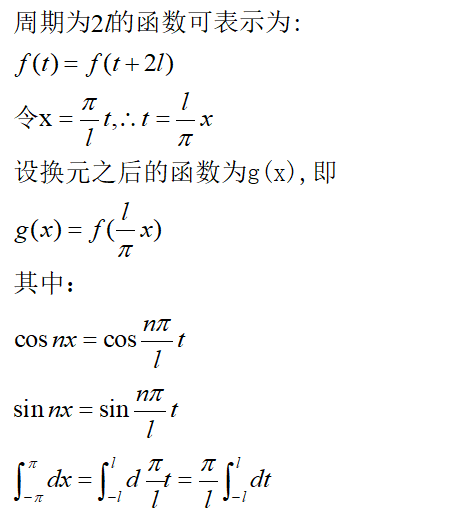由周期为2π的傅里叶级数：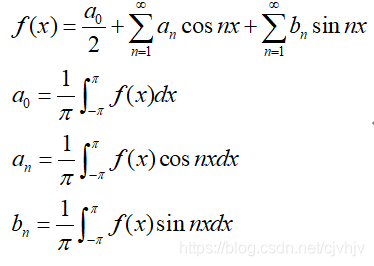可得周期为2L的傅里叶级数为：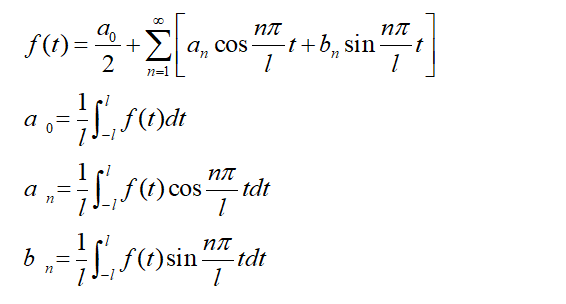四、周期函数傅里叶级数复数形式
引进复数形式最主要的目的是为了简化计算，在三角函数傅里叶级数展开中，有三个参数a0,an,bn，而通过复数形式，能够简化为一个参数Cn。而通过欧拉公式，就能从三角函数形式转变到复数形式；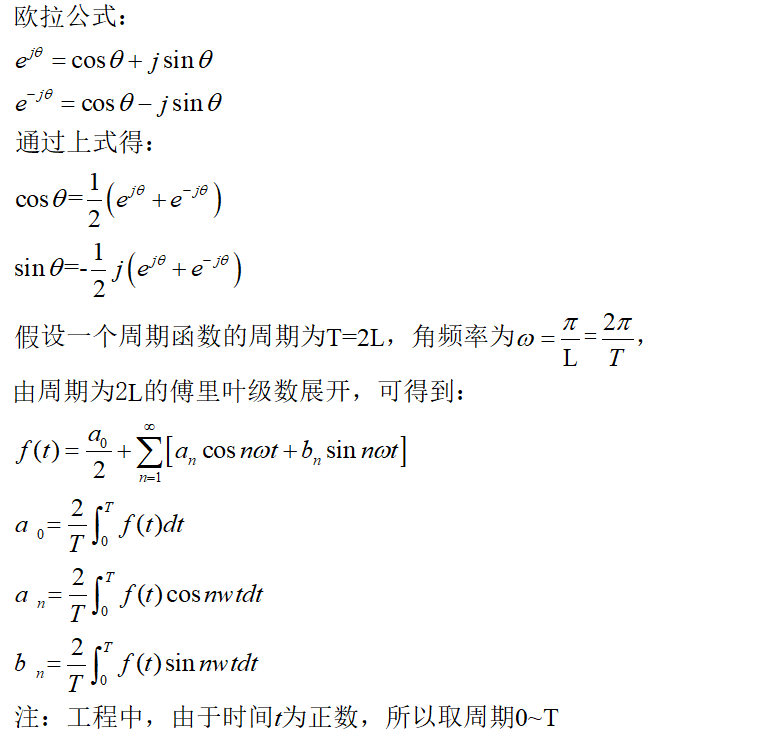通过欧拉公式可得：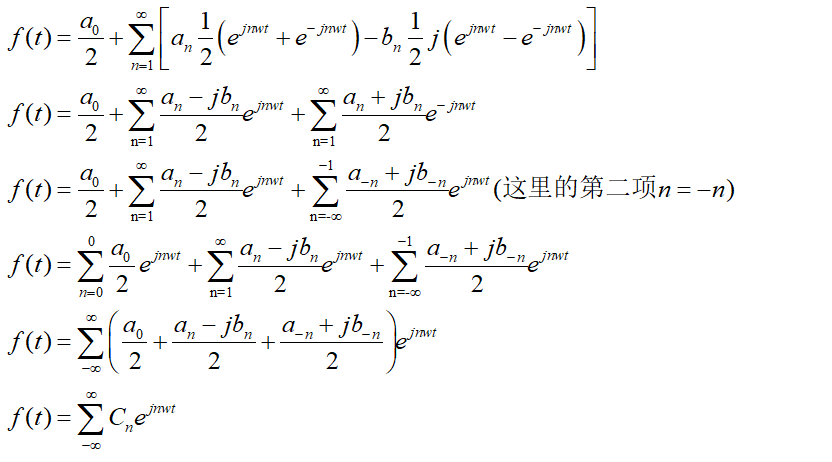求Cn: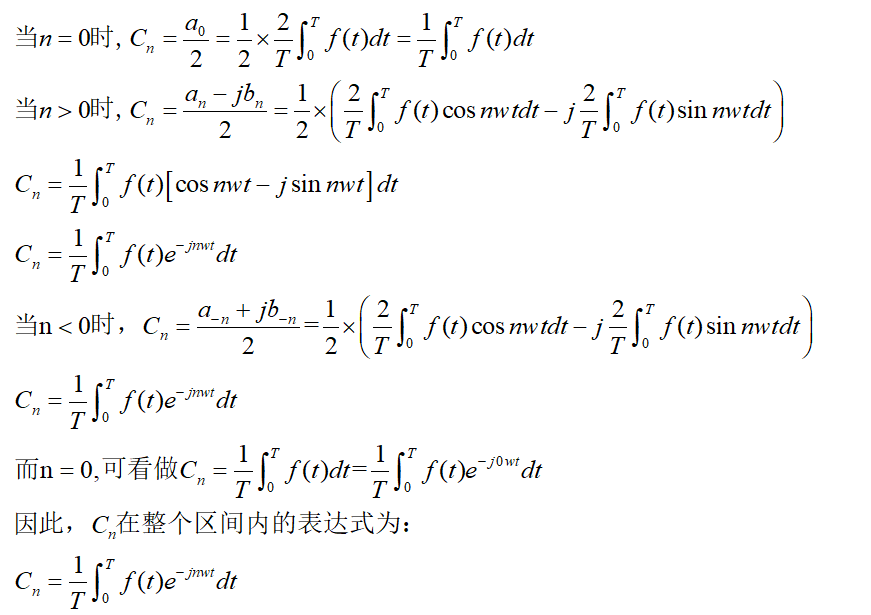所以傅里叶级数在复数表达的形式为：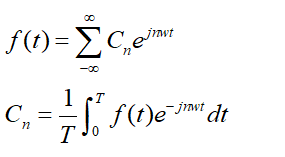由此可见，在复数的形式下，只有一个参数Cn，能够大大简化了计算
傅里叶变换公式推导（一）


展开全文经验分享
• 1、 考虑到一个函数可以展开成一个多项式的和，可惜多项式并不能直观的表示周期函数，由于正余弦函数是周期函数，可以考虑任意一个周期函数能否表示成为一系列正余弦函数的和。假设可以，不失一般性，于是得到： f...
• 相对论公式傅里叶级数展开的波函数离散解的本质分析和探讨，苏力宏，，本论文依据相对论方程的傅里叶级数表达式的波函数方程，对于引力场、量子力学和电磁方程进行了新的解释和应用，根据物质波理论对
• 傅里叶变换的由来及复数下的傅里叶变换公式证明 1、 考虑到一个函数可以展开成一个多项式的和，可惜多项式并不能直观的表示周期函数，由于正余弦函数是周期函数，可以考虑任意一个周期函数能否表示成为一系列正...
• 什么条件下函数可以展开傅里叶级数? 对于周期不是 2π2\pi2π的函数呢？ 傅里叶变换 能量谱密度 功率谱密度 参考文献 傅里叶变换表示形式 假设任意一个周期函数能否表示成为一系列正余弦函数的和： f(t)=A0+∑n=1...信号处理 fft
• 连续周期信号 x(t)=∑−∞+∞akejkw0t=∑−∞+∞akejk(2π/T)tak=1T∫Tx(t)e−jkw0tdt=1T∫Tx(t)e−jk(2π/T)tdtx(t)=∑−∞+∞akejkw0t=∑−∞+∞akejk(2π/T)tak=1T∫Tx(t)e−jkw0tdt=1T∫Tx(t)e−jk(2π/T)tdtx...
• 三、周期函数的傅里叶级数 由三角函数的正交性可知，一个周期函数可由完备正交函数集展开，即正交分解。假设有一个周期为2π的函数，那么它一定可以由前面的三角正交函数集分解，其形式为： 由上式可知，里面的未知...经验分享
• ## 幂级数展开公式

千次阅读 2018-08-20 09:25:46
• 1.为什么按照傅里叶公式做就可以将信号从时域转变到频域？ 2.为什么式中的e^(-jwt)部分会出现一个负号？有什么特定的意义？修改 举报1 条评论分享•邀请回答 按票数排序 17 个回答 匿名...
• 文章目录傅里叶级数信号的频率希尔伯特空间三角函数正交性推导简谐波构成的函数系傅里叶展开傅里叶级数推导表示任意的连续周期函数Fourier 级数的物理含义离散频谱与频谱图参考 傅里叶级数 傅里叶级数是基于一个问题...信号处理 深度学习
• 傅里叶展开锯齿波形图 复制matlab代码即可运行 %---------------------------------------- syms t3 A y3 y4 t y5 yposition yposition2 y33 % t3=0:1:1000; A=0.06; y3=A/2; f=0.2; w0=2pif; for i = 1:2:9 y4(t)=...matlab
• 看到论坛有一个朋友提问为什么傅里叶变换可以将时域变为频域？ 这个问题真是问到了灵魂深处。 在这我只能简单讲讲我的理解，要深刻理解翻信号处理教科书是最好的方法。 1. 如何描述信号 我们常常用数学模型去抽象...
• 超硬核之傅里叶公式推导 1.三角函数系与正交性2.周期(2pi)函数的傅里叶展开(三角形式)3.找到周期函数傅里叶展开的系数结语 前言:再学习数学推导之前,popcorn建议读者感性的先去理解一下傅里叶分析,可以参考我的文章...线性代数 算法 抽象代数 矩阵
• ## 方波信号傅里叶级数展开

万次阅读 多人点赞 2020-04-07 17:30:06
周期信号可以进行傅里叶级数展开 在研究非周期信号的傅里叶变换之前 首先应掌握傅里叶级数的三种表述形式： 三角函数形式 谐波形式 指数形式 并根据定义式求出傅里叶系数： 以周期性的方波信号为例，掌握傅里叶...
• 公式 给定一个周期为的函数，那么它可以表示为无穷级数： 其中傅里叶系数为： 几种常见波形的傅里叶级数展开式 梯形波（奇函数） 傅里叶展开为： 脉冲波（偶函数） 傅里叶展开为： 方波（奇函数） 傅里叶...线性代数 矩阵
• 把做工程过程比较重要的一些代码段记录起来，下面的代码是关于C语言通过傅里叶展开式计算圆周率PI的代码。 #include <iostream> #include <math.h> using namespace std; int main(){ long double pi...代码
• 在上一篇《浅谈傅里叶1》中已经给出了欧拉公式的推导和三角函数和的表达式，虽然三角函数和就是傅里叶公式的表达形式，但是数学家们还是嫌这样的表达式过于累赘，于是试图偷懒，通过将实数平面的三角函数和投影到复...高等数学
• 欧拉公式傅里叶变换对我们现在生活的影响真的很大呀！欧拉公式将三角函数和实数函数(e为底的指数函数)互转桥梁。 因此可以把三角函数中复杂的三角变换问题转到较直观的代数运算，也能够把高次幂的正余弦函数...
• 傅里叶级数展开的定义 将一个周期信号分解为一个直流分量和一系列复指数信号分量之和的过程被称为傅里叶级数展开。 周期信号f(t)f(t)f(t)的傅里叶级数展开式为：f(t)=∑k=−∞∞ckejkw0tf(t)=\sum_{k=-\infin}^{\...信号处理
• 傅立叶级数的介绍我就不说了，自己也是应用为主，之前一直觉得很难懂，但最近通过自己编程实现了一些函数的傅立叶级数展开之后对傅立叶 级数展开的概念比较清楚了 (1)函数如下 函数图象如下：   代码： from...
• 周期函数的傅里叶级数展开周期函数 周期函数 周期函数表达式为： f(x) = f(x + kT) (k = 1,2,3…) 如果该周期函数满足狄利赫里条件，那么该周期可以展开傅里叶级数： f(t)=a02+∑n=1∞(a0cos⁡(nω1t)+bnsin...数据分析 信号处理
• 再说傅里叶级数前谈一下傅里叶级数收敛条件，也就是什么情况下，一个周期上可积的f（x）可以展开傅里叶级数。 设f（x）是周期为2pi的周期函数，如果他满足： 1，在一个周期内连续或只有有限个第一类间断点 2，...
• 周期信号的傅里叶级数展开 ，在连续信号条件下，其傅里叶级数对为 x ( t ) = ∑ k = − ∞ + ∞ a k e j k ω 0 t = ∑ k = − ∞ + ∞ a k e j k ( 2 π / T ) t (1) (1) x ( t ) = ∑ k = − ∞ + ∞ a k e j k...信号与系统
• 如果一个函数的傅里叶级数处处收敛于 f(x)f(x)f(x)，则称这个级数是这个函数的傅里叶展开式，即： f(x)=a02+∑n=1∞an(cos⁡nx+bnsin⁡nx),x∈[−π,π] f(x)=\frac{a_{0}}{2}+\sum_{n=1}^{\infin}a_{n}(\cos{nx}+b_......